## Parabolic Cylindrical CoordinatesA system of Curvilinear Coordinates. There are several different conventions for the orientation and designation of these coordinates. Arfken (1970) defines coordinatessuch that(1)(2)(3)

In this work, following Morse and Feshbach (1953), the coordinatesare used instead. In this convention, the traces of the coordinate surfaces of the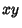-Plane are confocal Parabolas with a common axis. Thecurves open into the Negative x-Axis; the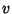curves open into the Positive x-Axis. Theandcurves intersect along the y-Axis.(4)(5)(6)

where,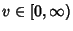, and. The Scale Factors are(7)(8)(9)

Laplace's Equation is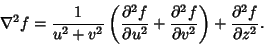(10)

The Helmholtz Differential Equation is Separable in parabolic cylindrical coordinates.

See also Confocal Paraboloidal Coordinates, Helmholtz Differential Equation--Parabolic Cylindrical Coordinates, Parabolic Coordinates

References

Arfken, G. Parabolic Cylinder Coordinates (,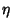,).'' §2.8 in Mathematical Methods for Physicists, 2nd ed. Orlando, FL: Academic Press, p. 97, 1970.

Morse, P. M. and Feshbach, H. Methods of Theoretical Physics, Part I. New York: McGraw-Hill, p. 658, 1953.